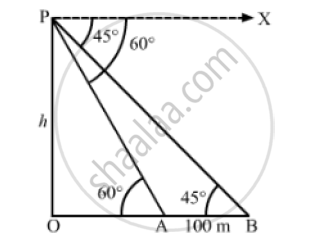# From the Top of a Vertical Tower, the Angles Depression of Two Cars in the Same Straight Line with the Base of the Tower, at an Instant Are Found to Be 45° and 60° . Find the Height of the Tower. - Mathematics

From the top of a vertical tower, the angles depression of two cars in the same straight line with the base of the tower, at an instant are found to be 45°  and 60° . If the cars are 100 m apart and are on the same side of the tower, find the height of the tower.

#### SolutionLet OP be the tower and points A and B be the positions of the cars.
We have,

AB =100m, ∠OAP = 60° and ∠OBP = 45°
Let OP = h
In ΔAOP,

 tan 60° = (OP)/(OA)

⇒ sqrt(3) = h/(OA)

⇒OA = h/sqrt(3)

Also, in Δ BOP ,

 tan 45° = (OP)/(OB)

⇒ 1 = h/(OB)

⇒ OB = h

Now,OB - OA = 100

⇒ h - h/sqrt(3) = 100

 ⇒ (h sqrt(3)-h)/sqrt(3) = 100

⇒(h(sqrt(3) -h))/sqrt(3) = 100

h = (100sqrt(3) )/((sqrt(3)-1)) xx ((sqrt(3)+1))/((sqrt(3)+1))

h = (100 sqrt(3) ( sqrt(3)+1))/((3-1))

⇒ h = (100(3+sqrt(3)))/2

⇒ h = 50(3+1.732)

⇒ h =50(4.732)

∴ h =236.6m

So, the height of the tower is 236.6 m.
Disclaimer. The answer given in the textbook is incorrect. The same has been rectified above.

Concept: Heights and Distances
Is there an error in this question or solution?
Chapter 14: Height and Distance - Exercises

#### APPEARS IN

RS Aggarwal Secondary School Class 10 Maths
Chapter 14 Height and Distance
Exercises | Q 31

Share